# Language Fundamentals OverView

Agenda :
1. Introduction
2. Identifiers
3. Reserved words
4. Data types
5. Literals
6. Arrays
7. Types of variables
8. Un initialized arrays
9. Var arg method
10. Main method
11. Command line arguments
12. Java coding standards
13. Various Memory areas present inside JVM

## Language Fundamentals

### Identifier :

A name in java program is called identifier. It may be class name, method name, variable name and label name.

Example: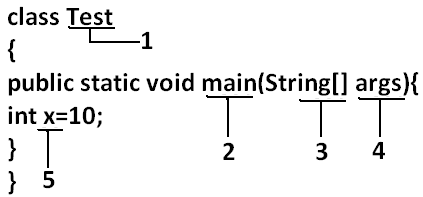### Rules to define java identifiers:

Rule 1: The only allowed characters in java identifiers are:
```1)	a to z
2)	A to Z
3)	0 to 9
4)	_ (underscore)
5)	\$
```
Rule 2: If we are using any other character we will get compile time error.
Example:
```1)	total_number-------valid
2)	Total#------------------invalid
```
Rule 3: identifiers are not allowed to starts with digit.
Example:
```1)	ABC123---------valid
2)	123ABC---------invalid
```
Rule 4: java identifiers are case sensitive up course java language itself treated as case sensitive language.
Example:
```class Test{
int number=10;
int Number=20;
int NUMBER=20;	we can differentiate with case.
int NuMbEr=30;
}
```
Rule 5: There is no length limit for java identifiers but it is not recommended to take more than 15 lengths.

Rule 6: We can't use reserved words as identifiers.
Example:
``` int if=10; --------------invalid
```
Rule 7: All predefined java class names and interface names we use as identifiers.

Example 1:
```class Test
{
public static void main(String[] args){
int String=10;
System.out.println(String);
}}
Output:
10
```

Example 2:
```class Test
{
public static void main(String[] args){
int Runnable=10;
System.out.println(Runnable);
}}
Output:
10
```

Even though it is legal to use class names and interface names as identifiers but it is not a good programming practice.

Which of the following are valid java identifiers?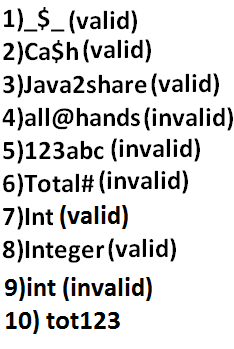### Reserved words:

In java some identifiers are reserved to associate some functionality or meaning such type of reserved identifiers are called reserved words.

Diagram: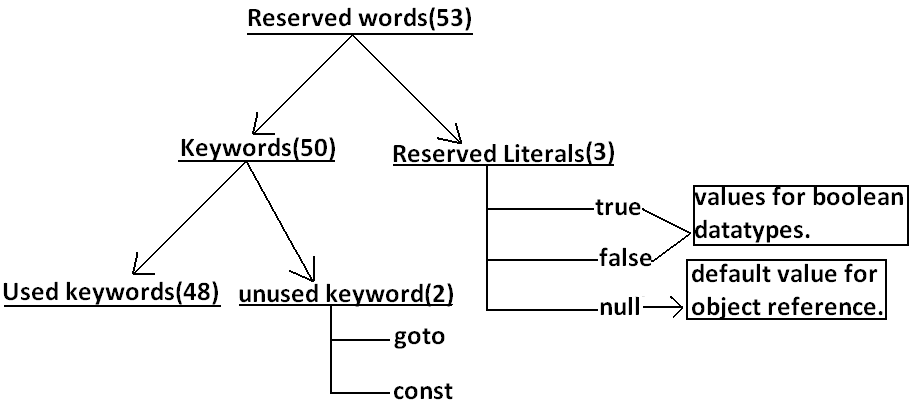### Reserved words for data types: (8)

```1)	byte
2)	short
3)	int
4)	long
5)	float
6)	double
7)	char
8)	boolean
```

### Reserved words for flow control:(11)

```1)	if
2)	else
3)	switch
4)	case
5)	default
6)	for
7)	do
8)	while
9)	break
10)	continue
11)	return
```

### Keywords for modifiers:(11)

```1)	public
2)	private
3)	protected
4)	static
5)	final
6)	abstract
7)	synchronized
8)	native
9)	strictfp(1.2 version)
10)	transient
11)	volatile
```

### Keywords for exception handling:(6)

```1)	try
2)	catch
3)	finally
4)	throw
5)	throws
6)	assert(1.4 version)
```

### Class related keywords:(6)

```1)	class
2)	package
3)	import
4)	extends
5)	implements
6)	interface
```

### Object related keywords:(4)

```1)	new
2)	instanceof
3)	super
4)	this
```

### Void return type keyword:

If a method won't return anything compulsory that method should be declared with the void return type in java but it is optional in C++.
```1)	void
```

### Unused keywords:

goto: Create several problems in old languages and hence it is banned in java.
Const: Use final instead of this.
By mistake if we are using these keywords in our program we will get compile time error.

### Reserved literals:

```1)	true	values for boolean data type.
2)	false
3)	null----------------- default value for object reference.
```

### Enum:

This keyword introduced in 1.5v to define a group of named constants
```Example:
enum Beer
{
KF, RC, KO, FO;
}
```

### Conclusions :

1. All reserved words in java contain only lowercase alphabet symbols.
2. New keywords in java are:
```strictfp-----------1.2v
assert-------------1.4v
enum--------------1.5v
```
3. In java we have only new keyword but not delete because destruction of useless objects is the responsibility of Garbage Collection.
4. ```instanceof  but not  instanceOf
strictfp    but not  strictFp
const      but not  Constant
syncronized but not  syncronize
extends      but not  extend
implements  but not  implement
import      but not  imports
int          but not  Int
```

### Which of the following list contains only java reserved words ?

1. final, finally, finalize (invalid) //here finalize is a method in Object class.
2. throw, throws, thrown(invalid) //thrown is not available in java
3. break, continue, return, exit(invalid) //exit is not reserved keyword
4. goto, constant(invalid) //here constant is not reserved keyword
5. byte, short, Integer, long(invalid) //here Integer is a wrapper class
6. extends, implements, imports(invalid) //imports keyword is not available in java
7. finalize, synchronized(invalid) //finalize is a method in Object class
8. instanceof, sizeOf(invalid) //sizeOf is not reserved keyword
9. new, delete(invalid) //delete is not a keyword
10. None of the above(valid)

### Which of the following are valid java keywords?

1. public(valid)
2. static(valid)
3. void(valid)
4. main(invalid)
5. String(invalid)
6. args(invalid)

### Data types:

Every variable has a type, every expression has a type and all types are strictly define more over every assignment should be checked by the compiler by the type compatibility hence java language is considered as strongly typed programming language.

### Java is pure object oriented programming or not?

Java is not considered as pure object oriented programming language because several oops features (like multiple inheritance, operator overloading) are not supported by java moreover we are depending on primitive data types which are non objects.

Diagram: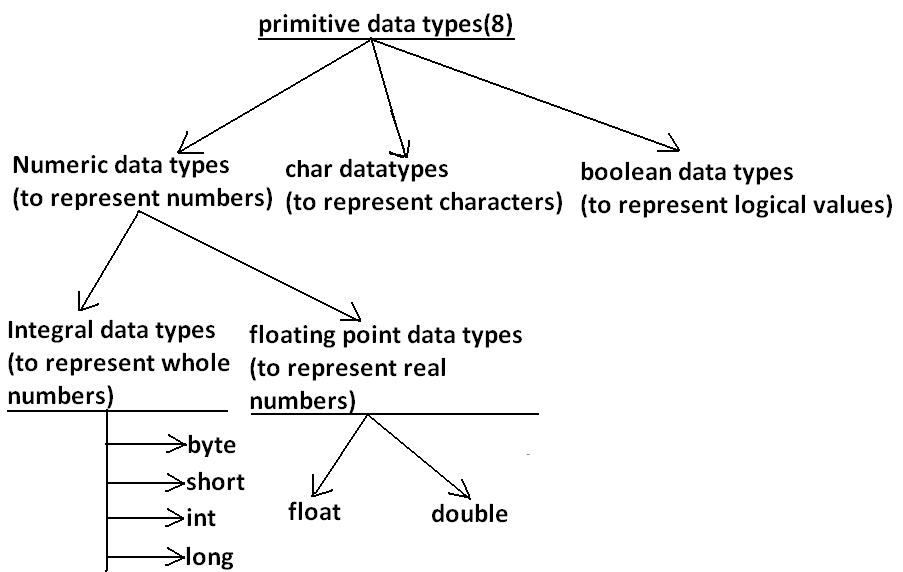Except Boolean and char all remaining data types are considered as signed data types because we can represent both "+ve" and"-ve" numbers.

### Byte:

```Size: 1byte (8bits)
Maxvalue: +127
Minvalue:-128
Range:-128to 127[-27 to 27-1]
```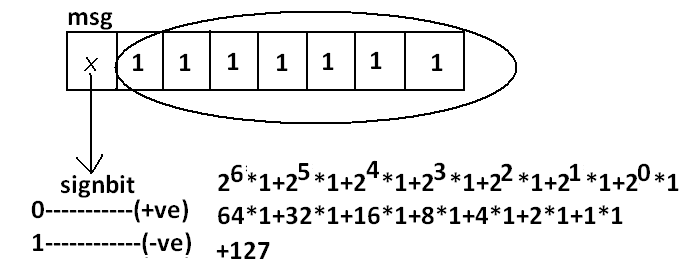• The most significant bit acts as sign bit. "0" means "+ve" number and "1" means "–ve" number.
• "+ve" numbers will be represented directly in the memory whereas "–ve" numbers will be represented in 2's complement form.
```Example:
byte b=10;
byte b2=130;//C.E:possible loss of precision
found : int
required : byte
byte b=10.5;//C.E:possible loss of precision
byte b=true;//C.E:incompatible types
byte b="ashok";//C.E:incompatible types
found : java.lang.String
required : byte
```
byte data type is best suitable if we are handling data in terms of streams either from the file or from the network.

### Short:

The most rarely used data type in java is short.
```Size: 2 bytes
Range: -32768 to 32767(-215 to 215-1)
```
Example:
```short s=130;
short s=32768;//C.E:possible loss of precision
short s=true;//C.E:incompatible types
```
Short data type is best suitable for 16 bit processors like 8086 but these processors are completely outdated and hence the corresponding short data type is also out data type.

### Int:

This is most commonly used data type in java.
```Size: 4 bytes
Range:-2147483648 to 2147483647 (-231 to 231-1)
```
Example:
```int i=130;
int i=10.5;//C.E:possible loss of precision
int i=true;//C.E:incompatible types
```

### long:

Whenever int is not enough to hold big values then we should go for long data type.
Example:
To hold the no. Of characters present in a big file int may not enough hence the return type of length() method is long.
```long l=f.length();//f is a file
Size: 8 bytes
Range:-263  to 263-1
```
Note: All the above data types (byte, short, int and long) can be used to represent whole numbers. If we want to represent real numbers then we should go for floating point data types.

### Floating Point Data types:

Floatdouble
If we want to 5 to 6 decimal places of accuracy then we should go for float. If we want to 14 to 15 decimal places of accuracy then we should go for double.
Size:4 bytes. Size:8 bytes.
Range:-3.4e38 to 3.4e38. -1.7e308 to1.7e308.
float follows single precision. double follows double precision.

### boolean data type:

```Size: Not applicable (virtual machine dependent)
Range: Not applicable but allowed values are true or false.
```

#### Which of the following boolean declarations are valid?

Example 1:
```boolean b=true;
boolean b=True;//C.E:cannot find symbol
boolean b="True";//C.E:incompatible types
boolean b=0;//C.E:incompatible types
```
Example 2:### Char data type:

In old languages like C & C++ are ASCII code based the no.Of ASCII code characters are < 256 to represent these 256 characters 8 - bits enough hence char size in old languages 1 byte.

In java we are allowed to use any worldwide alphabets character and java is Unicode based and no.Of unicode characters are > 256 and <= 65536 to represent all these characters one byte is not enough compulsory we should go for 2 bytes.
```Size: 2 bytes
Range: 0 to 65535
```
Example:
```char ch1=97;
char ch2=65536;//C.E:possible loss of precision
```

### Summary of java primitive data type:

data typeSizeRangeCorresponding Wrapper classDefault value
byte1 byte-27 to 27-1(-128 to 127) Byte0
short2 bytes-215 to 215-1 (-32768 to 32767)Short0
int 4 bytes -231 to 231-1 (-2147483648 to 2147483647) Integer 0
long 8 bytes -263 to 263-1 Long0
float 4 bytes -3.4e38 to 3.4e38 Float 0.0
double 8 bytes -1.7e308 to 1.7e308 Double 0.0
boolean Not applicable Not applicable(but allowed values true|false) Boolean false
char 2 bytes 0 to 65535 Character 0(represents blank space)

The default value for the object references is "null".

### Literals:

Any constant value which can be assigned to the variable is called literal.
Example:### Integral Literals:

For the integral data types (byte, short, int and long) we can specify literal value in the following ways.

1) Decimal literals: Allowed digits are 0 to 9.
Example: int x=10;

2) Octal literals: Allowed digits are 0 to 7. Literal value should be prefixed with zero.
Example: int x=010;

3) Hexa Decimal literals:
• The allowed digits are 0 to 9, A to Z.
• For the extra digits we can use both upper case and lower case characters.
• This is one of very few areas where java is not case sensitive.
• Literal value should be prefixed with ox(or)oX.
Example: int x=0x10;

These are the only possible ways to specify integral literal.

#### Which of the following are valid declarations?

1. int x=0777; //(valid)
2. int x=0786; //C.E:integer number too large: 0786(invalid)
3. int x=0xFACE; (valid)
4. int x=0xbeef; (valid)
5. int x=0xBeer; //C.E:';' expected(invalid) //:int x=0xBeer; ^// ^
6. int x=0xabb2cd;(valid)

Example:
```int x=10;
int y=010;
int z=0x10;
System.out.println(x+"----"+y+"----"+z); //10----8----16
```
By default every integral literal is int type but we can specify explicitly as long type by suffixing with small "l" (or) capital "L".

Example:
```int x=10;(valid)
long l=10L;(valid)
long l=10;(valid)
int x=10l;//C.E:possible loss of precision(invalid)
found : long
required : int
```
There is no direct way to specify byte and short literals explicitly. But whenever we are assigning integral literal to the byte variables and its value within the range of byte compiler automatically treats as byte literal. Similarly short literal also.

Example:
```byte b=127;(valid)
byte b=130;//C.E:possible loss of precision(invalid)
short s=32767;(valid)
short s=32768;//C.E:possible loss of precision(invalid)
```

### Floating Point Literals:

Floating point literal is by default double type but we can specify explicitly as float type by suffixing with f or F.

Example:
```float f=123.456;//C.E:possible loss of precision(invalid)
float f=123.456f;(valid)
double d=123.456;(valid)
```
We can specify explicitly floating point literal as double type by suffixing with d or D.

Example:
```double d=123.456D;
```
We can specify floating point literal only in decimal form and we can't specify in octal and hexadecimal forms.

Example:
```double d=123.456;(valid)
double d=0123.456;(valid)  //it is treated as decimal value  but not octal
double d=0x123.456;//C.E:malformed floating point literal(invalid)
```

#### Which of the following floating point declarations are valid?

1. float f=123.456; //C.E:possible loss of precision(invalid)
2. float f=123.456D; //C.E:possible loss of precision(invalid)
3. double d=0x123.456; //C.E:malformed floating point literal(invalid)
4. double d=0xFace; (valid)
5. double d=0xBeef; (valid)
We can assign integral literal directly to the floating point data types and that integral literal can be specified in decimal , octal and Hexa decimal form also.

Example:
```double d=0xBeef;
System.out.println(d);//48879.0
```
But we can't assign floating point literal directly to the integral types.

Example:
```int x=10.0;//C.E:possible loss of precision
```
We can specify floating point literal even in exponential form also(significant notation).

Example:
```double d=10e2;//==>10*102(valid)
System.out.println(d);//1000.0
float f=10e2;//C.E:possible loss of precision(invalid)
float f=10e2F;(valid)
```

### Boolean literals:

The only allowed values for the boolean type are true (or) false where case is important.
i.e., lower case

Example:
1. boolean b=true;(valid)
2. boolean b=0;//C.E:incompatible types(invalid)
3. boolean b=True;//C.E:cannot find symbol(invalid)
4. boolean b="true";//C.E:incompatible types(invalid)### Char literals:

1) A char literal can be represented as single character within single quotes.

Example:
1. char ch='a';(valid)
2. char ch=a;//C.E:cannot find symbol(invalid)
3. char ch="a";//C.E:incompatible types(invalid)
4. char ch='ab';//C.E:unclosed character literal(invalid)
2) We can specify a char literal as integral literal which represents Unicode of that character.
We can specify that integral literal either in decimal or octal or hexadecimal form but allowed values range is 0 to 65535.

Example:
1. char ch=97; (valid)
2. char ch=0xFace; (valid)
System.out.println(ch); //?
3. char ch=65536; //C.E: possible loss of precision(invalid)
3) We can represent a char literal by Unicode representation which is nothing but ‘\uxxxx' (4 digit hexa-decimal number) .

Example:
1. char ch='\ubeef';
2. char ch1='\u0061';
System.out.println(ch1); //a
3. char ch2=\u0062; //C.E:cannot find symbol
4. char ch3='\iface'; //C.E:illegal escape character
5. Every escape character in java acts as a char literal.

Example:
```1) char ch='\n';  //(valid)
2) char ch='\l';  //C.E:illegal escape character(invalid)
```
Escape CharacterDescription
\n New line
\t Horizontal tab
\r Carriage return
\f Form feed
\b Back space character
\' Single quote
\" Double quote
\\ Back space

#### Which of the following char declarations are valid?

1. char ch=a; //C.E:cannot find symbol(invalid)
2. char ch='ab'; //C.E:unclosed character literal(invalid)
3. char ch=65536; //C.E:possible loss of precision(invalid)
4. char ch=\uface; //C.E:illegal character: \64206(invalid)
5. char ch='/n'; //C.E:unclosed character literal(invalid)
6. none of the above. (valid)

### String literals:

Any sequence of characters with in double quotes is treated as String literal.

Example:
String s="Ashok"; (valid)

### 1.7 Version enhansements with respect to Literals :

The following 2 are enhansements
1. Binary Literals
2. Usage of '_' in Numeric Literals

### Binary Literals :

For the integral data types untill 1.6v we can specified literal value in the following ways
1. Decimal
2. Octal
3. Hexa decimal
But from 1.7v onwards we can specified literal value in binary form also.
The allowed digits are 0 to 1.
Literal value should be prefixed with Ob or OB .
```int x = 0b111;
System.out.println(x);  // 7
```

### Usage of _ symbol in numeric literals :

From 1.7v onwards we can use underscore(_) symbol in numeric literals.
```double d = 123456.789;  //valid

double d = 1_23_456.7_8_9;  //valid
double d = 123_456.7_8_9;   //valid
```
The main advantage of this approach is readability of the code will be improved At the time of compilation ' _ ' symbols will be removed automatically , hence after compilation the above lines will become double d = 123456.789

We can use more than one underscore symbol also between the digits.
Ex : double d = 1_23_ _456.789;

We should use underscore symbol only between the digits
```double d=_1_23_456.7_8_9;    //invalid
double d=1_23_456.7_8_9_;    //invalid
double d=1_23_456_.7_8_9;    //invalid
```
```double d='a';
System.out.println(d); //97
integral  data types
```
```float f=10L;
System.out.println(f); //10.0
floating-point  data types
```

Diagram: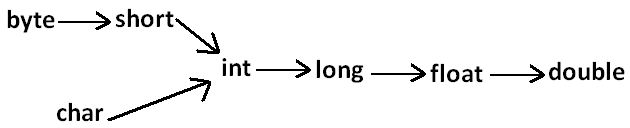### Arrays

1) Introduction
2) Array declaration
3) Array construction
4) Array initialization
5) Array declaration, construction, initialization in a single line.
6) length Vs length() method
7) Anonymous arrays
8) Array element assignments
9) Array variable assignments.

#### Introduction

An array is an indexed collection of fixed number of homogeneous data elements.

The main advantage of arrays is we can represent multiple values with the same name so that readability of the code will be improved.

But the main disadvantage of arrays is:
Fixed in size that is once we created an array there is no chance of increasing or decreasing the size based on our requirement that is to use arrays concept compulsory we should know the size in advance which may not possible always.

We can resolve this problem by using collections.

### Array declarations:

#### Single dimensional array declaration:

Example:
```int[] a;//recommended to use because name is clearly separated from the type
int []a;
int a[];
```
At the time of declaration we can't specify the size otherwise we will get compile time error.

Example:
```int[] a;//valid
int a;//invalid
```

#### Two dimensional array declaration:

Example:
```int[][] a;
int [][]a;
int a[][];      All are valid.(6 ways)
int[] []a;
int[] a[];
int []a[];
```

#### Three dimensional array declaration:

Example:
```int[][][] a;
int [][][]a;
int a[][][];
int[] [][]a;
int[] a[][];      All are valid.(10 ways)
int[] []a[];
int[][] []a;
int[][] a[];
int []a[][];
int [][]a[];
```
Which of the following declarations are valid?
```1)	int[] a1,b1; //a-1,b-1 (valid)
2)	int[] a2[],b2; //a-2,b-1 (valid)
3)	int[] []a3,b3; //a-2,b-2 (valid)
4)	int[] a,[]b; //C.E:<identifier> expected (invalid)
```
Note :
If we want to specify the dimension before the variable that rule is applicable only for the 1st variable.
Second variable onwards we can't apply in the same declaration.

Example:### Array construction:

Every array in java is an object hence we can create by using new operator.

Example:
```int[] a=new int;
```
Diagram:For every array type corresponding classes are available but these classes are part of java language and not available to the programmer level.

Array Type corresponding class name
int[] [I
int[][][[I
double[] [D

Rule 1:
At the time of array creation compulsory we should specify the size otherwise we will get compile time error.

Example:
```int[] a=new int;
int[] a=new int[];//C.E:array dimension missing
```
Rule 2:
It is legal to have an array with size zero in java.

Example:
```int[] a=new int;
System.out.println(a.length);//0
```
Rule 3:
If we are taking array size with -ve int value then we will get runtime exception saying NegativeArraySizeException.

Example:
```int[] a=new int[-3];//R.E:NegativeArraySizeException
```
Rule 4:
The allowed data types to specify array size are byte, short, char, int.
By mistake if we are using any other type we will get compile time error.

Example:
```int[] a=new int['a'];//(valid)
byte b=10;
int[] a=new int[b];//(valid)
short s=20;
int[] a=new int[s];//(valid)
int[] a=new int[10l];//C.E:possible loss of precision//(invalid)
int[] a=new int[10.5];//C.E:possible loss of precision//(invalid)
```
Rule 5:
The maximum allowed array size in java is maximum value of int size .

Example:
```int[] a1=new int;(valid)
int[] a2=new int;
//C.E:integer number too large: 2147483648(invalid)
```
In the first case we may get RE : OutOfMemoryError.

#### Multi dimensional array creation:

In java multidimensional arrays are implemented as array of arrays approach but not matrix form.
The main advantage of this approach is to improve memory utilization.

Example 1:
```int[][] a=new int[];
a=new int;
a=new int;
```
Diagram:Example 2:
```int[][][] a=new int[][];
a=new int[];
a=new int;
a=new int;
a=new int;
a=new int;
```
Diagram: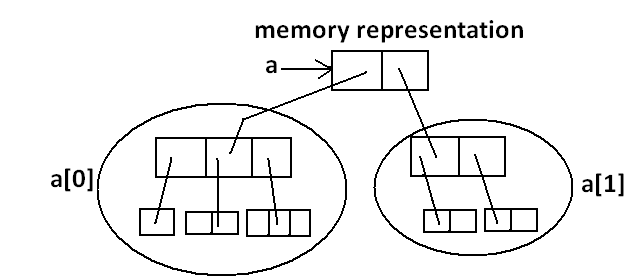Which of the following declarations are valid?
```1)	int[] a=new int[]//C.E: array dimension missing(invalid)
2)	int[][] a=new int;(valid)
3)	int[][] a=new int[];(valid)
4)	int[][] a=new int[];//C.E:']' expected(invalid)
5)	int[][][] a=new int;(valid)
6)	int[][][] a=new int[];(valid)
7)	int[][][] a=new int[];//C.E:']' expected(invalid)
```

### Array Initialization:

Whenever we are creating an array every element is initialized with default value automatically.

Example 1:
```int[] a=new int;
System.out.println(a);//[I@3e25a5
System.out.println(a);//0
```
Diagram: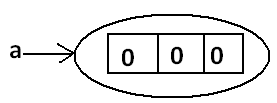Note: Whenever we are trying to print any object reference internally toString() method will be executed which is implemented by default to return the following. classname@hexadecimalstringrepresentationofhashcode.

Example 2: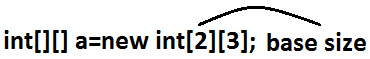```System.out.println(a);//[[I@3e25a5
System.out.println(a);//[I@19821f
System.out.println(a);//0
```
Diagram: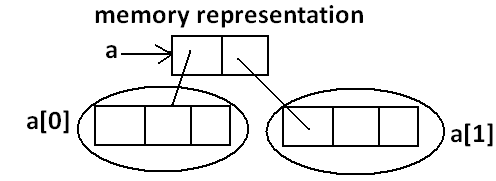Example 3:
```int[][] a=new int[];
System.out.println(a);//[[I@3e25a5
System.out.println(a);//null
System.out.println(a);//R.E:NullPointerException
```
Diagram: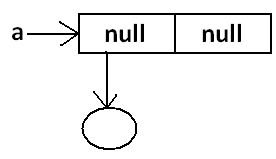Once we created an array all its elements by default initialized with default values.
If we are not satisfied with those default values then we can replays with our customized values.

Example:
```int[] a=new int;
a=10;
a=20;
a=30;
a=40;
a=50;//R.E:ArrayIndexOutOfBoundsException: 4
a[-4]=60;//R.E:ArrayIndexOutOfBoundsException: -4
```
Diagram:Note: if we are trying to access array element with out of range index we will get Runtime Exception saying ArrayIndexOutOfBoundsException.

### Declaration, construction and initialization of an array in a single line:

We can perform declaration, construction and initialization of an array in a single line.

Example: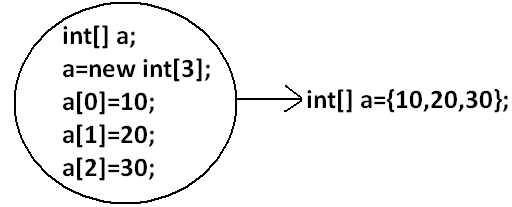```char[] ch={'a','e','i','o','u'};(valid)
String[] s={"balayya","venki","nag","chiru"};(valid)
```
We can extend this short cut even for multi dimensional arrays also.

Example:
```int[][] a={{10,20,30},{40,50}};
```
Diagram: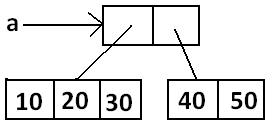Example:
```int[][][] a={{{10,20,30},{40,50}},{{60},{70,80},{90,100,110}}};
```
Diagram: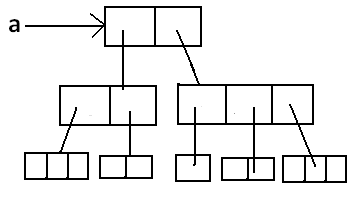```int[][][] a={{{10,20,30},{40,50}},{{60},{70,80},{90,100,110}}};
System.out.println(a);//50(valid)
System.out.println(a);//R.E:ArrayIndexOutOfBoundsException: 2(invalid)
System.out.println(a);//100(valid)
System.out.println(a);//110(valid)
System.out.println(a);//R.E:ArrayIndexOutOfBoundsException: 2(invalid)
System.out.println(a);//80(valid)
```
• If we want to use this short cut compulsory we should perform declaration, construction and initialization in a single line.
• If we are trying to divide into multiple lines then we will get compile time error.

Example: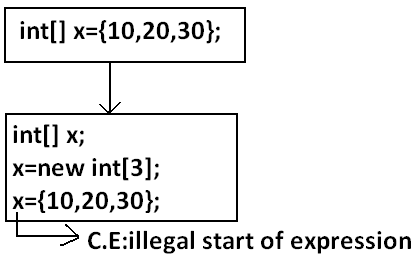### length Vs length():

#### length:

1. It is the final variable applicable only for arrays.
2. It represents the size of the array.
Example:
```int[] x=new int;
System.out.println(x.length());//C.E: cannot find symbol
System.out.println(x.length);//3
```

#### length() method:

1. It is a final method applicable for String objects.
2. It returns the no of characters present in the String.
Example:
```String s="bhaskar";
System.out.println(s.length);//C.E:cannot find symbol
System.out.println(s.length());//7
```
In multidimensional arrays length variable represents only base size but not total size.

Example:
```int[][] a=new int;
System.out.println(a.length);//6
System.out.println(a.length);//3
```
Diagram: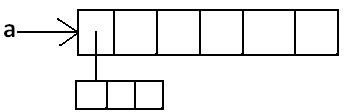length variable applicable only for arrays where as length()method is applicable for String objects.

There is no direct way to find total size of multi dimentional array but indirectly we can find as follows
x[o].length +x.length + x.length + .......

### Anonymous Arrays:

• Sometimes we can create an array without name such type of nameless arrays are called anonymous arrays.
• The main objective of anonymous arrays is "just for instant use".
• We can create anonymous array as follows.
```new int[]{10,20,30,40};(valid)
new int[][]{{10,20},{30,40}};(valid)
```
• At the time of anonymous array creation we can't specify the size otherwise we will get compile time error.
Example:
```new int{10,20,30,40};//C.E:';' expected(invalid)
new int[]{10,20,30,40};(valid)
```
Based on our programming requirement we can give the name for anonymous array then it is no longer anonymous.

Example:
`int[] a=new int[]{10,20,30,40};(valid)  `
Example:
```class Test
{
public static void main(String[] args)
{
System.out.println(sum(new int[]{10,20,30,40}));//100
}
public static int sum(int[] x)
{
int total=0;
for(int x1:x)
{
total=total+x1;
}
}
}
```
In the above program just to call sum() , we required an array but after completing sum() call we are not using that array any more, ananimous array is best suitable.

### Array element assignments:

Case 1:
In the case of primitive array as array element any type is allowed which can be promoted to declared type.

Example 1:
For the int type arrays the allowed array element types are byte, short, char, int.
```int[] a=new int;
a=97;//(valid)
a='a';//(valid)
byte b=10;
a=b;//(valid)
short s=20;
a=s;//(valid)
a=10l;//C.E:possible loss of precision
```
Example 2:
For float type arrays the allowed element types are byte, short, char, int, long, float.Case 2:
In the case of Object type arrays as array elements we can provide either declared type objects or its child class objects.
Example 1:
```Object[] a=new Object;
a=new Integer(10);//(valid)
a=new Object();//(valid)
```
Example 2:
```Number[] n=new Number;
n=new Integer(10);//(valid)
n=new Double(10.5);//(valid)
```
Diagram: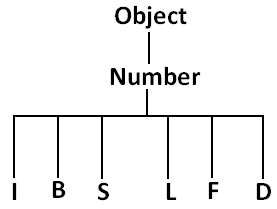Case 3:
In the case of interface type arrays as array elements we can provide its implemented class objects.

Example:
```Runnable[] r=new Runnable;
```
Array TypeAllowed Element Type
1) Primitive arrays.1) Any type which can be promoted to declared type.
2) Object type arrays.2) Either declared type or its child class objects allowed.
3) Interface type arrays.3) Its implemented class objects allowed.
4) Abstract class type arrays.4) Its child class objects are allowed.

### Array variable assignments:

Case 1:
• Element level promotions are not applicable at array object level.
• Ex : A char value can be promoted to int type but char array cannot be promoted to int array.
Example:
```int[] a={10,20,30};
char[] ch={'a','b','c'};
int[] b=a;//(valid)
int[] c=ch;//C.E:incompatible types(invalid)
```
Which of the following promotions are valid?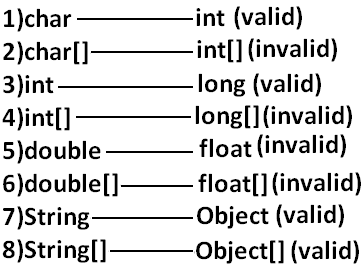Note: In the case of object type arrays child type array can be assign to parent type array variable.

Example:
```String[] s={"A","B"};
Object[] o=s;
```
Case 2:
Whenever we are assigning one array to another array internal elements won't be copy just reference variables will be reassigned hence sizes are not important but types must be matched.

Example:
```int[] a={10,20,30,40,50,60,70};
int[] b={80,90};
a=b;//(valid)
b=a;//(valid)
```
Diagram:Case 3:
Whenever we are assigning one array to another array dimensions must be matched that is in the place of one dimensional array we should provide the same type only otherwise we will get compile time error.

Example:
```int[][] a=new int[];
a=new int;//C.E:incompatible types(invalid)
a=10;//C.E:incompatible types(invalid)
a=new int;//(valid)
```
Note: Whenever we are performing array assignments the types and dimensions must be matched but sizes are not important.

Example 1:
```int[][] a=new int;
a=new int;
a=new int;
a=new int;
```
Diagram: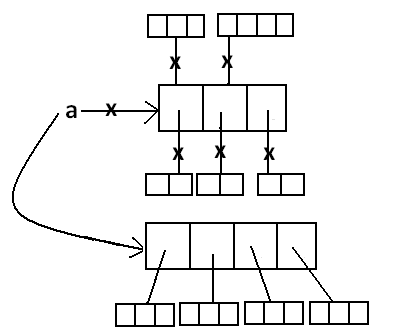Total how many objects created?
Ans: 11
How many objects eligible for GC: 6

Example 2:
```class Test
{
public static void main(String[] args)
{
String[] argh={"A","B"};
args=argh;
System.out.println(args.length);//2
for(int i=0;i<=args.length;i++)
{
System.out.println(args[i]);
}
}
}
Output:
java Test x y
R.E: ArrayIndexOutOfBoundsException: 2
java Test x
R.E: ArrayIndexOutOfBoundsException: 2
java Test
R.E: ArrayIndexOutOfBoundsException: 2
```
Note: Replace with i<args.length

Example 3:
```class Test
{
public static void main(String[] args)
{
String[] argh={"A","B"};
args=argh;
System.out.println(args.length);//2
for(int i=0;i<args.length;i++)
{
System.out.println(args[i]);
}
}
}
Output:
2
A
B
```

Example 4:
```class Test
{
public static void main(String[] args)
{
String[] argh={"A","B"};
args=argh;

for(String s : args) {
System.out.println(s);
}
}
Output:
A
B
```

### Types of Variables

Division 1 : Based on the type of value represented by a variable all variables are divided into 2 types. They are:
1. Primitive variables
2. Reference variables

#### Primitive variables:

Primitive variables can be used to represent primitive values.

Example: int x=10;

#### Reference variables:

Reference variables can be used to refer objects.

Example: Student s=new Student();

Diagram:Division 2 : Based on the behaviour and position of declaration all variables are divided into the following 3 types.
1. Instance variables
2. Static variables
3. Local variables

#### Instance variables:

• If the value of a variable is varied from object to object such type of variables are called instance variables.
• For every object a separate copy of instance variables will be created.
• Instance variables will be created at the time of object creation and destroyed at the time of object destruction hence the scope of instance variables is exactly same as scope of objects.
• Instance variables will be stored on the heap as the part of object.
• Instance variables should be declared with in the class directly but outside of any method or block or constructor.
• Instance variables can be accessed directly from Instance area. But cannot be accessed directly from static area.
• But by using object reference we can access instance variables from static area.
Example:
```class Test
{
int i=10;
public static void main(String[] args)
{
//System.out.println(i);
//C.E:non-static variable i cannot be referenced from a static context(invalid)
Test t=new Test();
System.out.println(t.i);//10(valid)
t.methodOne();
}
public void methodOne()
{
System.out.println(i);//10(valid)
}
}
```
For the instance variables it is not required to perform initialization JVM will always provide default values.

Example:
```class Test
{
boolean b;
public static void main(String[] args)
{
Test t=new Test();
System.out.println(t.b);//false
}
}
```
Instance variables also known as object level variables or attributes.

#### Static variables:

• If the value of a variable is not varied from object to object such type of variables is not recommended to declare as instance variables. We have to declare such type of variables at class level by using static modifier.
• In the case of instance variables for every object a separate copy will be created but in the case of static variables for entire class only one copy will be created and shared by every object of that class.
• Static variables will be crated at the time of class loading and destroyed at the time of class unloading hence the scope of the static variable is exactly same as the scope of the .class file.
• Static variables will be stored in method area. Static variables should be declared with in the class directly but outside of any method or block or constructor.
• Static variables can be accessed from both instance and static areas directly.
• We can access static variables either by class name or by object reference but usage of class name is recommended.
• But within the same class it is not required to use class name we can access directly.
java TEST
1. Start JVM.
2. Create and start Main Thread by JVM.
3. Locate(find) Test.class by main Thread.
5. Execution of main() method.
6. Unload Test.class     // static variable destruction
8. Shutdown JVM.

Example:
```class Test
{
static int i=10;
public static void main(String[] args)
{
Test t=new Test();
System.out.println(t.i);//10
System.out.println(Test.i);//10
System.out.println(i);//10
}
}
```
For the static variables it is not required to perform initialization explicitly, JVM will always provide default values.

Example:
```class Test
{
static String s;
public static void main(String[] args)
{
System.out.println(s);//null
}
}
```
Example:
```class Test
{
int x=10;
static int y=20;
public static void main(String[] args)
{
Test t1=new Test();
t1.x=888;
t1.y=999;
Test t2=new Test();
System.out.println(t2.x+"----"+t2.y);//10----999
}
}
```
Diagram:Static variables also known as class level variables or fields.

#### Local variables:

Some times to meet temporary requirements of the programmer we can declare variables inside a method or block or constructors such type of variables are called local variables or automatic variables or temporary variables or stack variables.

Local variables will be stored inside stack.

The local variables will be created as part of the block execution in which it is declared and destroyed once that block execution completes. Hence the scope of the local variables is exactly same as scope of the block in which we declared.

Example 1:
```class Test
{
public static void main(String[] args)
{
int i=0;
for(int j=0;j<3;j++)
{
i=i+j;
}}
}
```
Example 2:
```class Test
{
public static void main(String[] args)
{
try
{
int i=Integer.parseInt("ten");
}
catch(NullPointerException e)
{}
}
}
```
• The local variables will be stored on the stack.
• For the local variables JVM won't provide any default values compulsory we should perform initialization explicitly before using that variable.

Example:Example:
```class Test
{
public static void main(String[] args)
{
int x;
if(args.length>0)
{
x=10;
}
System.out.println(x);
//C.E:variable x might not have been initialized
}
}
```
Example:
```class Test
{
public static void main(String[] args)
{
int x;
if(args.length>0)
{
x=10;
}
else
{
x=20;
}
System.out.println(x);
}
}
Output:
java Test x
10
java Test x y
10
java Test
20
```
• It is never recommended to perform initialization for the local variables inside logical blocks because there is no guarantee of executing that block always at runtime.
• It is highly recommended to perform initialization for the local variables at the time of declaration at least with default values.
Note: The only applicable modifier for local variables is final. If we are using any other modifier we will get compile time error.

Example:
```class Test
{
public static void main(String[] args)
{

public int x=10; //(invalid)
private int x=10; //(invalid)
protected int x=10; //(invalid)    C.E: illegal start of expression
static int x=10; //(invalid)
volatile int x=10; //(invalid)
transient int x=10; //(invalid)

final int x=10;//(valid)
}
}
```

### Conclusions:

1. For the static and instance variables it is not required to perform initialization explicitly JVM will provide default values. But for the local variables JVM won't provide any default values compulsory we should perform initialization explicitly before using that variable.
2. For every object a separate copy of instance variable will be created whereas for entire class a single copy of static variable will be created. For every Thread a separate copy of local variable will be created.
3. Instance and static variables can be accessed by multiple Threads simultaneously and hence these are not Thread safe but local variables can be accessed by only one Thread at a time and hence local variables are Thread safe.
4. If we are not declaring any modifier explicitly then it means default modifier but this rule is applicable only for static and instance variables but not local variable.

### Un Initialized arrays

Example:
```class Test
{
int[] a;
public static void main(String[] args)
{
Test t1=new Test();
System.out.println(t1.a);//null
System.out.println(t1.a);//R.E:NullPointerException
}
}
```

#### Instance level:

Example 1:
```int[] a;
System.out.println(obj.a);//null
System.out.println(obj.a);//R.E:NullPointerException
```
Example 2:
```int[] a=new int;
System.out.println(obj.a);//[I@3e25a5
System.out.println(obj.a);//0
```

#### Static level:

Example 1:
```static int[] a;
System.out.println(a);//null
System.out.println(a);//R.E:NullPointerException
```
Example 2:
```static int[] a=new int;
System.out.println(a);//[I@3e25a5
System.out.println(a);//0
```

#### Local level:

Example 1:
```int[] a;
System.out.println(a); //C.E: variable a might not have been initialized
System.out.println(a);
```
Example 2:
```int[] a=new int;
System.out.println(a);//[I@3e25a5
System.out.println(a);//0
```
Once we created an array every element is always initialized with default values irrespective of whether it is static or instance or local array.

Every variable in java should be either instance or static or local.
Every variable in java should be either primitive or reference

Hence the following are the various possible combinations for variables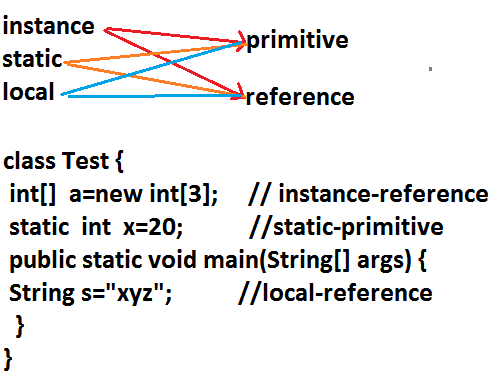### Var- arg methods (variable no of argument methods) (1.5)

• Until 1.4v we can't declared a method with variable no. Of arguments.
• If there is a change in no of arguments compulsory we have to define a new method.
• This approach increases length of the code and reduces readability.
• But from 1.5 version onwards we can declare a method with variable no. Of arguments such type of methods are called var-arg methods.
We can declare a var-arg method as follows.We can call or invoke this method by passing any no. Of int values including zero number also.

Example:
```class Test
{
public static void methodOne(int... x)
{
System.out.println("var-arg method");
}
public static void main(String[] args)
{
methodOne();
methodOne(10);
methodOne(10,20,30);
}
}
Output:
var-arg method
var-arg method
var-arg method
```
Internally var-arg parameter implemented by using single dimensional array hence within the var-arg method we can differentiate arguments by using index.

Example:
```class Test
{
public static void sum(int... x)
{
int total=0;
for(int i=0;i<x.length;i++)
{
total=total+x[i];
}
System.out.println("The sum :"+total);
}
public static void main(String[] args)
{
sum();
sum(10);
sum(10,20);
sum(10,20,30,40);
}
}
Output:
The sum: 0
The sum: 10
The sum: 30
The sum: 100
```

Example:
```class Test
{
public static void sum(int... x)
{
int total=0;
for(int x1 : x)
{
total=total+x1;
}
System.out.println("The sum :"+total);
}
public static void main(String[] args)
{
sum();
sum(10);
sum(10,20);
sum(10,20,30,40);
}
}
Output:
The sum: 0
The sum: 10
The sum: 30
The sum: 100
```

Case 1:
Which of the following var-arg method declarations are valid?
1. methodOne(int... x) (valid)
2. methodOne(int ...x) (valid)
3. methodOne(int...x) (valid)
4. methodOne(int x...) (invalid)
5. methodOne(int. ..x) (invalid)
6. methodOne(int .x..) (invalid)

Case 2:
We can mix var-arg parameter with general parameters also.

Example:
```methodOne(int a,int... b)        //valid
methodOne(String s,int... x)	//valid
```
Case 3:
if we mix var-arg parameter with general parameter then var-arg parameter should be the last parameter.

Example:
```methodOne(int a,int... b)        //valid
methodOne(int... a,int b)   //(invalid)
```
Case 4:
With in the var-arg method we can take only one var-arg parameter. i.e., if we are trying to more than one var-arg parameter we will get CE.

Example:
`methodOne(int... a,int... b)  //(invalid)`
Case 5:
```class Test
{
public static void methodOne(int i)
{
System.out.println("general method");
}
public static void methodOne(int... i)
{
System.out.println("var-arg method");
}
public static void main(String[] args)
{
methodOne();//var-arg method
methodOne(10,20);//var-arg method
methodOne(10);//general method
}
}
```
In general var-arg method will get least priority that is if no other method matched then only var-arg method will get the chance this is exactly same as default case inside a switch.

Case 6:
For the var-arg methods we can provide the corresponding type array as argument.

Example:
```class Test
{{
System.out.println("var-arg method");
}
public static void main(String[] args)
{
methodOne(new int[]{10,20,30});//var-arg method
}
}
```
Case 7:
```class Test
{
public void methodOne(int[] i){}
public void methodOne(int... i){}
}
Output:
Compile time error.
Cannot declare both methodOne(int...) and methodOne(int[]) in Test
```

### Single Dimensional Array Vs Var-Arg Method:

Case 1:
Wherever single dimensional array present we can replace with var-arg parameter.Example:
```class Test
{
public static void main(String... args)
{
System.out.println("var-arg main method");//var-arg main method
}
}
```

Case 2:
Wherever var-arg parameter present we can't replace with single dimensional array.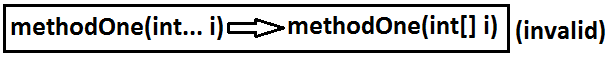Note :
1. methodOne(int... x)
we can call this method by passing a group of int values and x will become 1D array. (i.e., int[] x)
2. methodOne(int[]... x)
we can call this method by passing a group of 1D int[] and x will become 2D array. ( i.e., int[][] x)
Above reasons this case 2 is invalid. Example:
```class Test
{
public static void methodOne(int[]... x)
{
for(int[] a:x)
{
System.out.println(a);
}
}
public static void main(String[] args)
{
int[] l={10,20,30};
int[] m={40,50};
methodOne(l,m);

}
}
Output:
10
40
```
Analysis: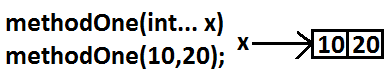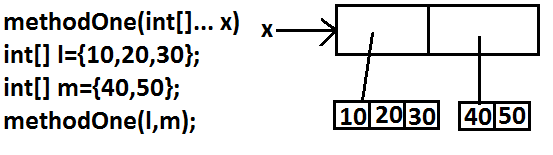### Main Method

Whether the class contains main() method or not,
and whether it is properly declared or not,
these checking's are not responsibilities of the compiler, at runtime JVM is responsible for this.
If JVM unable to find the required main() method then we will get runtime exception saying NoSuchMethodError: main.

Example:
```class Test
{}
Output:
javac Test.java
java Test R.E: NoSuchMethodError: main
```
At runtime JVM always searches for the main() method with the following prototype.If we are performing any changes to the above syntax then the code won't run and will get Runtime exception saying NoSuchMethodError.

Even though above syntax is very strict but the following changes are acceptable to main() method.
1. The order of modifiers is not important that is instead of public static we can take static public.
2. We can declare string[] in any acceptable form
• String[] args
• String []args
• String args[]
3. Instead of args we can use any valid java identifier.
4. We can replace string[] with var-arg parameter.
Example: main(String... args)
5. main() method can be declared with the following modifiers.
final, synchronized, strictfp.
```class Test {
static final syncronized strictfp public void  main(String... ask){
System.out.println("valid  main method");
}
}
output :
valid main method
```

#### Which of the following main() method declarations are valid ?

1. public static void main(String args){} (invalid)
2. public synchronized final strictfp void main(String[] args){} (invalid)
3. public static void Main(String... args){} (invalid)
4. public static int main(String[] args){} //int return type we can't take //(invalid)
5. public static synchronized final strictfp void main(String... args){}(valid)
6. public static void main(String... args){}(valid)
7. public void main(String[] args){}(invalid)

#### In which of the above cases we will get compile time error ?

No case, in all the cases we will get runtime exception.

Case 1 :
Overloading of the main() method is possible but JVM always calls string[] argument main() method only.

Example:
```class Test
{
public static void main(String[] args)
{
System.out.println("String[] array main method");      //overloaded methods
}
public static void main(int[] args)
{
System.out.println("int[] array main method");
}
}
Output:
String[] array main method
```

The other overloaded method we have to call explicitly then only it will be executed.

Case 2:
Inheritance concept is applicable for static methods including main() method
hence while executing child class if the child class doesn't contain main() method then the parent class main() method will be executed.

Example 1:
```class Parent
{
public static void main(String[] args)
{
System.out.println("parent main");          //Parent.java
}
}
class Child extends Parent
{}
```
Analysis: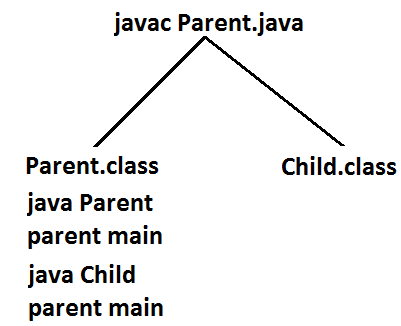Example 2:
```class Parent
{
public static void main(String[] args)
{
System.out.println("parent main");         // Parent.java
}
}
class Child extends Parent
{
public static void main(String[] args)
{
System.out.println("Child main");
}
}
```
Analysis: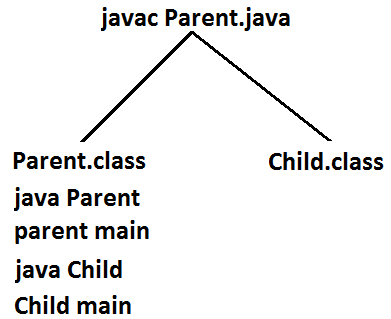It seems to be overriding concept is applicable for static methods but it is not overriding it is method hiding.

### 1.7 Version Enhansements with respect to main() :

Case 1 :
• Untill 1.6v if our class doesn't contain main() method then at runtime we will get Runtime Exception saying NosuchMethodError:main
• But from 1.7 version onwards instead of NoSuchMethodError we will get more meaning full description
```class Test {
}

1.6 version :
javac Test.java
java Test
RE: NoSuchMethodError:main

1.7 version :
javac Test.java
java Test
public static void main(String[]  args)
```

Case 2 :
From 1.7 version onwards to start program execution compulsory main method should be required, hence even though the class contains static block if main method not available then won't be executed
```class Test {
static {
System.out.println("static block");
}
}

1.6 version :
javac Test.java
java Test
output :
static block
RE: NoSuchMethodError:main

1.7 version :
javac Test.java
java Test
public static void main(String[]  args)
```
Case 3 :
```class Test {
static {
System.out.println("static block");
System.exit(0);
}
}

1.6 version :
javac Test.java
java Test
output :
static block

1.7 version :
javac Test.java
java Test
public static void main(String[]  args)
```
Case 4 :
```class Test {
static {
System.out.println("static block");
}
public static void main(String[] args) {
System.out.println("main method");
}
}

1.6 version :
javac Test.java
java Test
output :
static block
main method

1.7 version :
javac Test.java
java Test
output :
static block
main method
```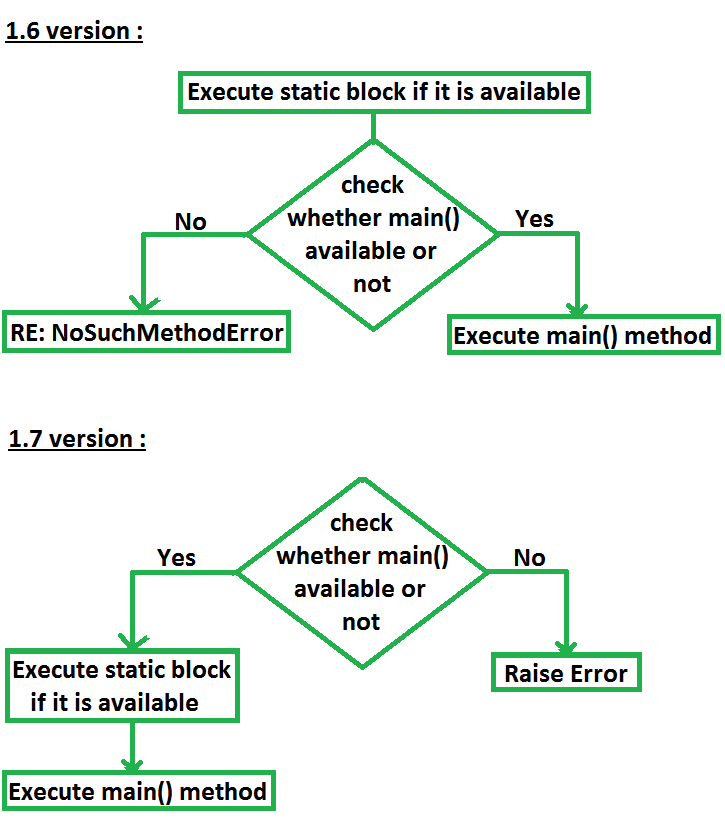### Command line arguments:

The arguments which are passing from command prompt are called command line arguments.
The main objective of command line arguments are we can customize the behavior of the main() method.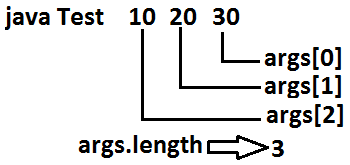Example 1:
```class Test
{
public static void main(String[] args)
{
for(int i=0;i<=args.length;i++)
{
System.out.println(args[i]);
}
}
}
Output:
java Test x y z
ArrayIndexOutOfBoundsException: 3
```
Replace i<=args.length with i<args.length then it will run successfully.

Example 2 :
```class Test
{
public static void main(String[] args)
{
String[] argh={"X","Y","Z"};
args=argh;
for(String s : args)
{
System.out.println(s);
}
}
}
Output:
java Test A B C
X
Y
Z

java Test A B
X
Y
Z

java Test
X
Y
Z
```

Within the main() method command line arguments are available in the form of String
hence "+" operator acts as string concatenation but not arithmetic addition.

Example 3 :
```class Test
{
public static void main(String[] args)
{
System.out.println(args+args);
}
}
Output:
E:\SCJP>javac Test.java
E:\SCJP>java Test 10 20
1020
```
Space is the separator between 2 command line arguments and if our command line argument itself contains space then we should enclose with in double quotes.

Example 4 :
```class Test
{
public static void main(String[] args)
{
System.out.println(args);
}
}
Output:
E:\SCJP>javac Test.java
E:\SCJP>java Test "Sai Charan"
Sai Charan
```

### Java coding standards

• Whenever we are writing java code , It is highly recommended to follow coding standards , which improves the readability and understandability of the code.
• Whenever we are writing any component(i.e., class or method or variable) the name of the component should reflect the purpose or functionality.
Example: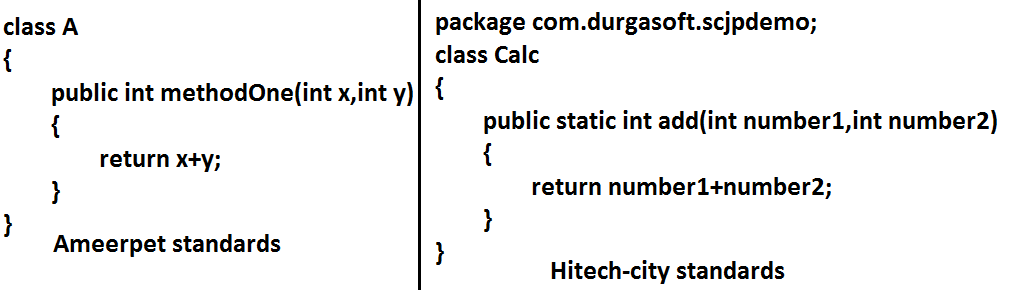#### Coding standards for classes:

• Usually class names are nouns.
• Should starts with uppercase letter and if it contains multiple words every inner word should starts with upper case letter.

Example: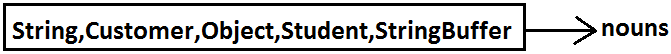#### Coding standards for interfaces:

• Usually interface names are adjectives.
• Should starts with upper case letter and if it contains multiple words every inner word should starts with upper case letter.

Example:
1. Serializable
2. Runnable
3. Cloneable

#### Coding standards for methods:

• Usually method names are either verbs or verb-noun combination.
• Should starts with lowercase character and if it contains multiple words every inner word should starts with upper case letter.(camel case convention)

Example: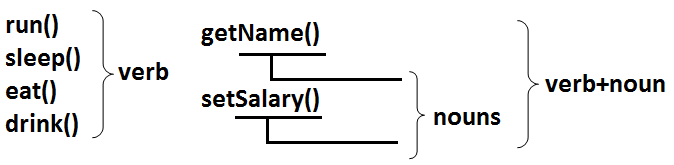#### Coding standards for variables:

• Usually variable names are nouns.
• Should starts with lowercase alphabet symbol and if it contains multiple words every inner word should starts with upper case character.(camel case convention)

Example:
```length
name
salary            	        nouns
age
mobileNumber
```

#### Coding standards for constants:

• Usually constants are nouns.
• Should contain only uppercase characters and if it contains multiple words then these words are separated with underscore symbol.
• Usually we can declare constants by using public static final modifiers.

Example:
```MAX_VALUE
MIN_VALUE                           nouns
NORM_PRIORITY
```

#### Java bean coding standards:

A java bean is a simple java class with private properties and public getter and setter methods.

Example:#### Syntax for setter method:

1. Method name should be prefixed with set.
2. It should be public.
3. Return type should be void.
4. Compulsory it should take some argument.

#### Syntax for getter method:

1. The method name should be prefixed with get.
2. It should be public.
3. Return type should not be void.
4. It is always no argument method.
Note: For the boolean properties the getter method can be prefixed with either get or is.
But recommended to use is.

Example: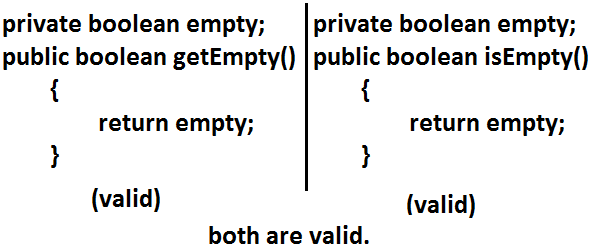### Coding standards for listeners:

#### To register a listener:

Method name should be prefixed with add.
1. public void addMyActionListener(MyActionListener l) (valid)
2. public void registerMyActionListener(MyActionListener l) (invalid)
3. public void addMyActionListener(ActionListener l) (invalid)

#### To unregister a listener:

The method name should be prefixed with remove.
1. public void removeMyActionListener(MyActionListener l) (valid)
2. publc void unregisterMyActionListener(MyActionListener l) (invalid)
3. public void removeMyActionListener(ActionListener l) (invalid)
4. public void deleteMyActionListener(MyActionListener l) (invalid)

### Various Memory areas present inside JVM :1. Class level binary data includung static variables will be stored in method area.
2. Objects and corresponding instance variables will be stored in Heap area.
3. For every method the JVM will create a Runtime stack all method calls performed by that Thread and corresponding local variables will be stored in that stack.
Every entry in stack is called Stack Frame or Action Record.
4. The instruction which has to execute next will be stored in the corresponding PC Registers.
5. Native method invocations will be stored in native method stacks.
 BACK